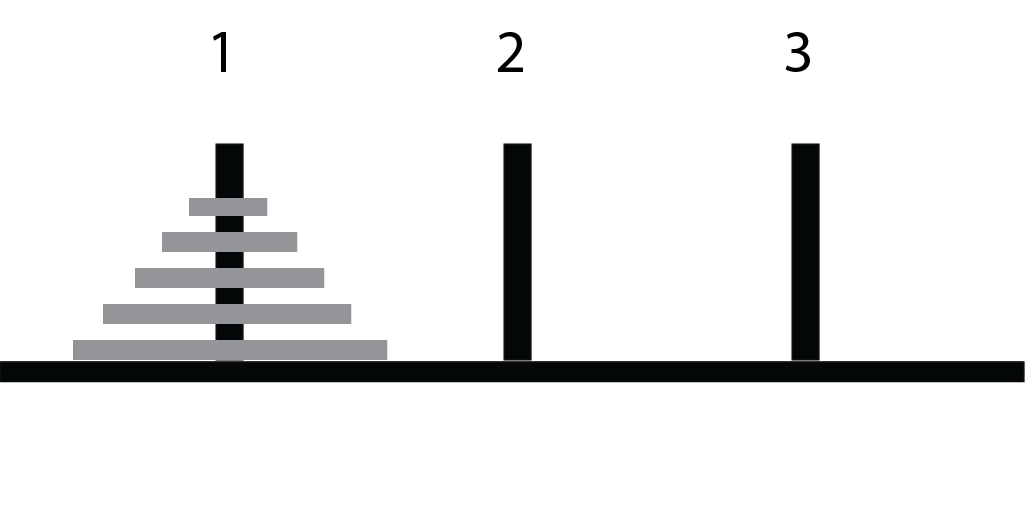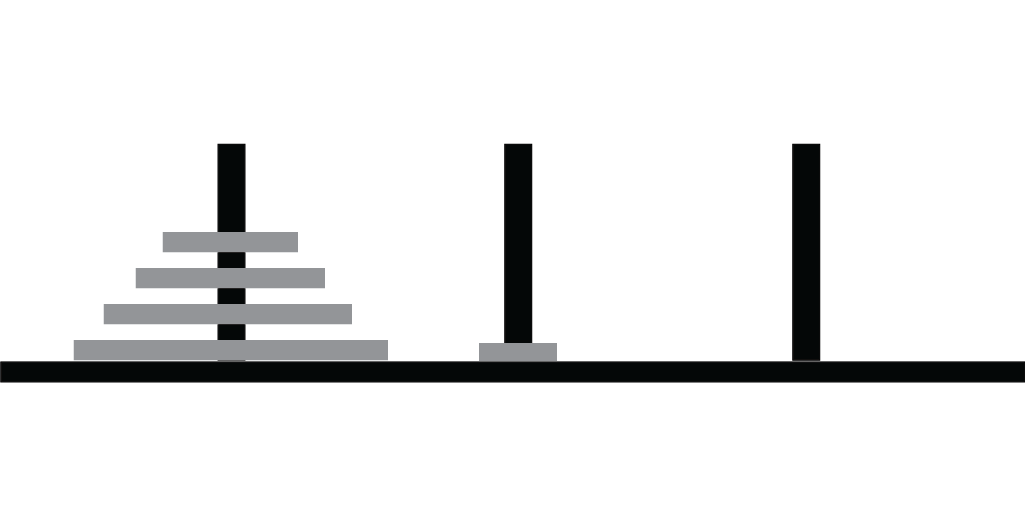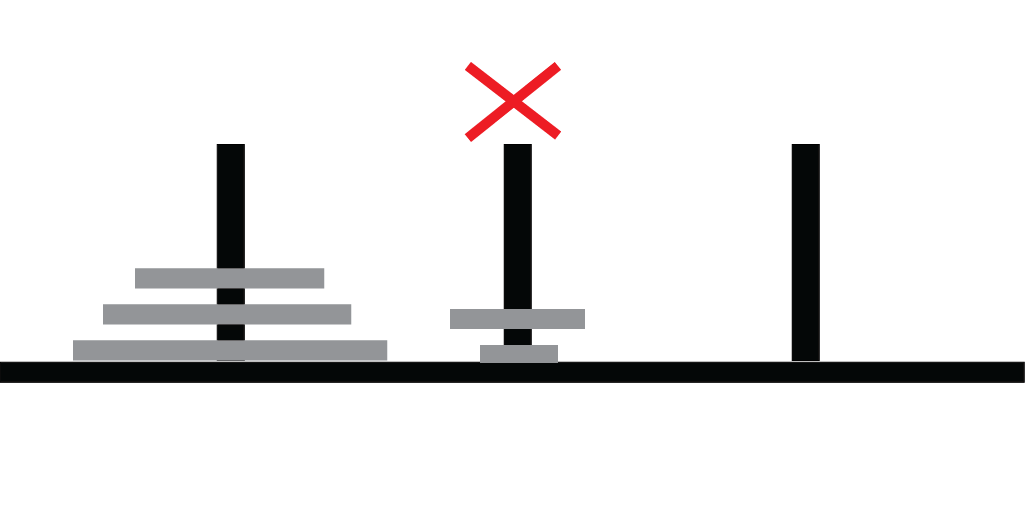# Lecture 04: Recursion III

## Outline

1. Tower of Hanoi
• recursive strategy
• in code
2. Concluding Thoughts on Recursion
3. Preview: Defining New Objects

## Setup## Goal## Rules## A Program

Want:

• A program that generates instructions for a solution
• Example solution for 2 disks
Move disk from peg 1 to peg 2
Move disk from peg 1 to peg 3
Move disk from peg 2 to peg 3


## Moving the Largest Disk

How to move largest disk from peg 1 to peg 3?

## Solving in 3 Steps (1)

Step 1: Move subtower to peg 2## Solving in 3 Steps (2)

Step 2: Move bottom disk to peg 3## Solving in 3 Steps (3)

Step 3: Move subtower to peg 3## Moving a Sub-Tower

How do we move $$m$$ disks from peg $$i$$ to peg $$j$$?

## A General Procedure

To move $$m$$ disks from peg $$i$$ to $$j$$:

1. move $$m - 1$$ disks from $$i$$ to $$k$$ (other peg)
2. move one disk from $$i$$ to $$j$$
3. move $$m - 1$$ disks from $$k$$ to $$j$$

What is missing?

## Base Case!

If $$m = 1$$, just print

Move disk from peg i to peg j


## Let’s try it in code!

TowerOfHanoi.java

## How Efficient is our Solution?

void move (int m, int from, int to, int other) {
if (m == 1) {
System.out.println("Move disk from " + from + " to " + to);
return;
}

move(m - 1, from, other, to);
move(1, from, to, other);
move(m - 1, other, to, from);
}


## Counting Recursive Calls

If $$f(m )$$ is number of instructions printed to move $$m$$ disks:

• $$f(m) = 2 \cdot f(m-1) + f(1)$$, and $$f(1) = 1$$
• So we get:
• $f(1) = 1$
• $f(2) = 2 \cdot f(1) + 1 = 3$
• $f(3) = 2 \cdot f(2) + 1 = 7$
• $\vdots$
• $f(m) = 2^m - 1$
• This is a lot of instructions!
• $f(10) \approx 1,000$, $f(20) \approx 1,000,000$, $f(30) \approx 1,000,000,000$

## But…

Solving Tower of Hanoi with $m$ disks requires $2^m - 1$ instructions!

• The solution generated by our program is optimal
• Requires only 5 lines of code!

# Concluding Thoughts on Recursion

## Factorial

private static int factorial(int n) {
if (n == 1) return 1;
return n * factorial(n - 1);
}


## Fibonacci

private static int fibonacci (int n) {
if (n <= 2) return 1;
return fibonacci(n - 1) + fibonacci(n - 2);
}


## Tower of Hanoi

private static void move (int num, int from, int to, int other) {
if (num == 1) {
System.out.println("Move disk from " + from + " to " + to + "." );
return;
}
move(num - 1, from, other, to);
move(1, from, to, other);
move(num - 1, other, to, from);
}


## Evaluation

1. Factorial: simple code and efficient execution
2. Fibonacci: simple code, but inefficient execution
3. ToH: surprisingly simple; perhaps mysterious; large solution
• but solution is still optimal

## Moral

Recursion is…

• subtle
• sometimes efficient, sometimes not
• powerful
• miraculous
• confusing

## How Confusing?

public static long collatz (long n) {
if (n == 1) return 1;
if (n % 2 == 0) return collatz (n / 2);
else return collatz (3 * n + 1);
}


## Insanity

public static long collatz (long n) {
if (n == 1) return 1;
if (n % 2 == 0) return collatz (n / 2);
else return collatz (3 * n + 1);
}

• It is not known if this method has an infinite loop for some value of n
• This is not for lack of interest in the problem:
• Worked on by some of the most celebrated mathematicians of the last century